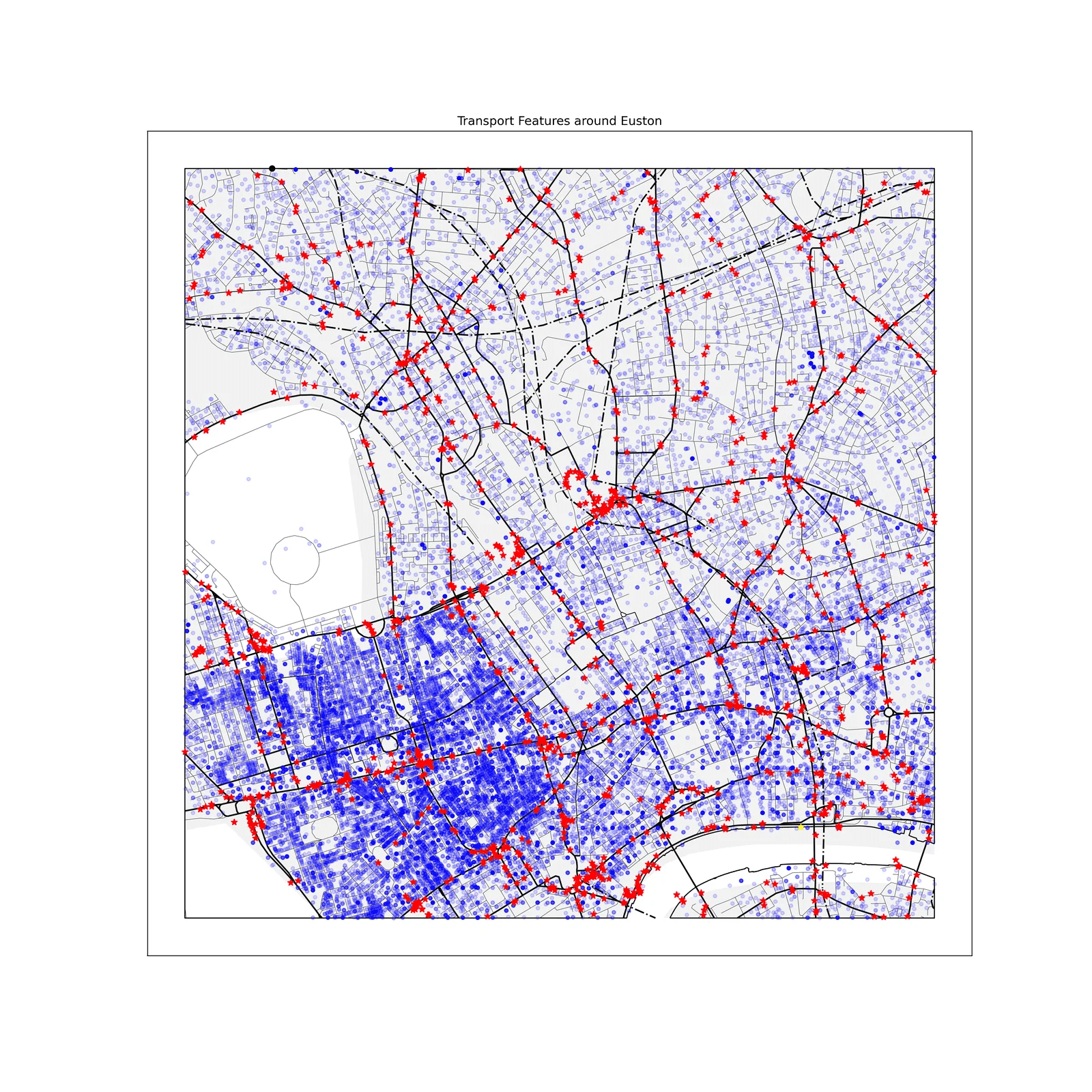# Nearest neighbour speed tests

In my last post “How far is it to the nearest train station?” I shared some code to find the nearest neighbour for each point in a geopandas dataframe to each point in another geopandas dataframe. The bethod involved using the balltree function from sklearn. I want to generalise this method so that, for a given set of candidate points, we can find the nearest geographic item of interest. This item could be a point (e.g. train station) line (e.g. a road) or polygon e.g. a national park.

I’ve so far tried and failed to generalise the previous function to work for any geometry item. I decided to brute force it using a much simpler function. How much worse can it be?

## New function

``````def distance(src_points, candidates, source_name, out_name, keep_d_n_g=[True,True]):

dist_name = "Distance_To_Nearest_" + out_name + "(m)"
name_name = "Name_of_Nearest_" + out_name
geom_name = "Geometry_of_Nearest_" + out_name

candidates = candidates.reset_index(drop=True)

dist_df = src_points.geometry.apply(lambda g: candidates.distance(g))
min_distance = list(dist_df.min(axis=1).round(0))
min_name = list(candidates[source_name].iloc[dist_df.idxmin(axis=1)])
min_geom = list(candidates["geometry"].iloc[dist_df.idxmin(axis=1)])

df = pd.DataFrame(list(zip(min_distance, min_name, min_geom)),
columns =[dist_name, name_name, geom_name],
index =src_points.index)

return df``````

For each source point we’re finding the distance to every candidate point and keeping the closest one. There are a lot of calculations happening here. It’s not converting to numpy arrays…etc. so I’m not expecting this to be quick. But, this does complete my brief of working on of the pairs point x {point, line, polygon} so in that sense it is better than the method from my previous post

## The Test

I’ll use the same data as in the previous post. Find the nearest train station to each postcode in (a) a 5km square around Euston station (b) the whole UK. And see which function performs the quickest.In the terms of this map, that is finding the nearest green star to each blue dot.

## Results

On the Euston data the brute force method takes 43ms and the balltree method takes 23ms. So that’s twice as long. So it seems better, but probably acceptable for small datasets.

How about the big dataset (2.7 million postcodes and 500+ train stations)?

The balltree method took 61s, so is very quick. I’ve left the brute force method running for over a couple and it still hasn’t finished. So it’s safe to say it’s definitely not as quick!

## Conclusion

The brute force method is fine for small datasets.

For anything big it’s not good at all. I’ll continue to look for a solution to make this search efficient.

All code for this project can be found on github https://github.com/richardkappa/LandReg_py